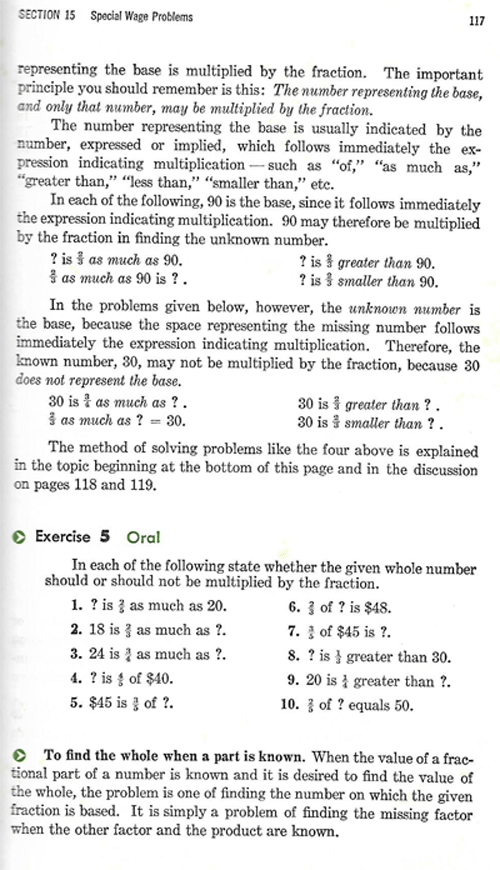SEARCH HOMEMath Central Quandaries & QueriesQuestion from Kenneth: Question: I have an old business mathematics textbook. The authors have indicated that the following expressions indicate multiplication: \$? is 2/3 greater than 90; ? is 2/3 smaller than 90. They also indicated that the following expression would indicate division: 30 is 2/3 greater than ? and 30 is 2/3 smaller than ?. How can these phrases indicate multiplication and division? How can 60 be 2/3 greater than 90 and also smaller than 90 as indicated above. What were the authors thinking? I have added the page from the book that indicates what I have explained in my message I thank you for any helpful reply that may be sent to me. KennethHi Kenneth,

I have added the page you sent me.I also find this disturbing. If I asked for ? where "? is a more than 90" and a = 5, then the answer would be ? = 95. But the author seems to be saying that if a is a fraction, for example, a = 2/3 then you multiply 90 by a rather than adding a to 90.

In the explanation the author says that "of", "as much as", "greater than", "less than", "smaller than", etc. all indicate multiplication. I completely disagree. "Of" and "as much as" indicate multiplication but "greater than", "less than", and "smaller than" have completely different meanings.

HarleyMath Central is supported by the University of Regina and The Pacific Institute for the Mathematical Sciences.